ZHCSEF5G May   2014  – September 2016

PRODUCTION DATA.

1. 特性
2. 应用
3. 说明
4. 修订历史记录
5. Pin Configuration and Functions
6. Specifications
7. Detailed Description
1. 7.1 Overview
2. 7.2 Functional Block Diagram
3. 7.3 Feature Description
4. 7.4 Device Functional Modes
8. Application and Implementation
1. 8.1 Application Information
2. 8.2 Typical Applications
1. 8.2.1 Standard Circuit
2. 8.2.2 Alternative Two-Wire Application
9. Power Supply Recommendations
10. 10Layout
11. 11器件和文档支持
1. 11.1 器件支持
2. 11.2 接收文档更新通知
3. 11.3 社区资源
4. 11.4 商标
5. 11.5 静电放电警告
6. 11.6 Glossary
12. 12机械、封装和可订购信息

• DBZ|3
• LPG|3

## 8 Application and Implementation

NOTE

Information in the following applications sections is not part of the TI component specification, and TI does not warrant its accuracy or completeness. TI’s customers are responsible for determining suitability of components for their purposes. Customers should validate and test their design implementation to confirm system functionality.

### 8.1 Application Information

The DRV5033 device is used in magnetic-field sensing applications.

### 8.2 Typical Applications

#### 8.2.1 Standard Circuit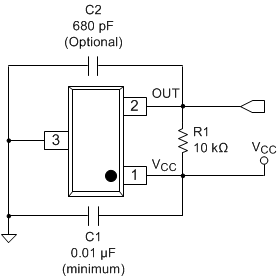Figure 18. Typical Application Circuit

#### 8.2.1.1 Design Requirements

For this design example, use the parameters listed in Table 2 as the input parameters.

### Table 2. Design Parameters

DESIGN PARAMETER REFERENCE EXAMPLE VALUE
Supply voltage VCC 3.2 to 3.4 V
System bandwidth ƒBW 10 kHz

### Table 3. External Components

COMPONENT PIN 1 PIN 2 RECOMMENDED
C1 VCC GND A 0.01-µF (minimum) ceramic capacitor rated for VCC
C2 OUT GND Optional: Place a ceramic capacitor to GND
R1 OUT REF(1) Requires a resistor pullup
REF is not a pin on the DRV5033 device, but a REF supply-voltage pullup is required for the OUT pin; the OUT pin may be pulled up to VCC.

#### 8.2.1.2.1 Configuration Example

In a 3.3-V system, 3.2 V ≤ Vref ≤ 3.4 V. Use Equation 3 to calculate the allowable range for R1.

Equation 3.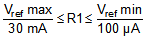For this design example, use Equation 4 to calculate the allowable range of R1.

Equation 4.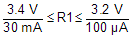Therefore:

Equation 5. 113 Ω ≤ R1 ≤ 32 kΩ

After finding the allowable range of R1 (Equation 5), select a value between 500 Ω and 32 kΩ for R1.

Assuming a system bandwidth of 10 kHz, use Equation 6 to calculate the value of C2.

Equation 6.For this design example, use Equation 7 to calculate the value of C2.

Equation 7.An R1 value of 10 kΩ and a C2 value less than 820 pF satisfy the requirement for a 10-kHz system bandwidth.

A selection of R1 = 10 kΩ and C2 = 680 pF would cause a low-pass filter with a corner frequency of 23.4 kHz.

#### 8.2.1.3 Application CurvesR1 = 10 kΩ pullup No C2
Figure 19. 10-kHz Switching Magnetic Field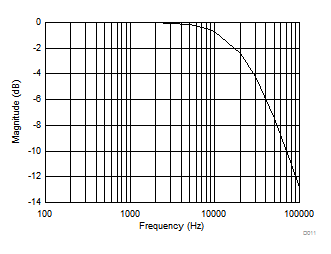R1 = 10-kΩ pullup C2 = 680 pF
Figure 21. Low-Pass FilteringR1 = 10-kΩ pullup C2 = 680 pF
Figure 20. 10-kHz Switching Magnetic Field

#### 8.2.2 Alternative Two-Wire Application

For systems that require minimal wire count, the device output can be connected to VCC through a resistor, and the total supplied current can be sensed near the controller.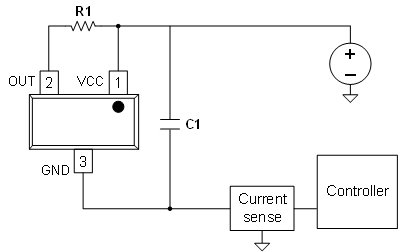Figure 22. 2-Wire Application

Current can be sensed using a shunt resistor or other circuitry.

#### 8.2.2.1 Design Requirements

Table 4 lists the related design parameters.

### Table 4. Design Parameters

DESIGN PARAMETER REFERENCE EXAMPLE VALUE
Supply voltage VCC 12 V
OUT resistor R1 1 kΩ
Bypass capacitor C1 0.1 µF
Current when B < BRP IRELEASE About 3 mA
Current when B > BOP IOPERATE About 15 mA

#### 8.2.2.2 Detailed Design Procedure

When the open-drain output of the device is high-impedance, current through the path equals the ICC of the device (approximately 3 mA).

When the output pulls low, a parallel current path is added, equal to VCC / (R1 + rDS(on)). Using 12 V and 1 kΩ, the parallel current is approximately 12 mA, making the total current approximately 15 mA.

The local bypass capacitor C1 should be at least 0.1 µF, and a larger value if there is high inductance in the power line interconnect.